# GMAT Math : Cubes

## Example Questions

← Previous 1

### Example Question #51 : Rectangular Solids & Cylinders

What is the length of the edge of a cube?

1) Its volume is 1,728 cubic meters.

2) Its surface area is 864 square meters

BOTH statements TOGETHER are sufficient, but neither statement ALONE is sufficient.

Statements 1 and 2 TOGETHER are not sufficient.

Statement 1 ALONE is sufficient, but Statement 2 alone is not sufficient.

EACH statement ALONE is sufficient.

Statement 2 ALONE is sufficient, but Statement 1 alone is not sufficient.

EACH statement ALONE is sufficient.

Explanation:

Call the sidelength, surface area, and volume of the cube,, and, respectively.

Then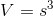or, equivalently,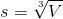So, given statement 1 alone - that is, given only the volume, you can demonstrate the sidelength to be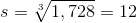Also,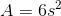or, equivalently,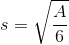Given statement 2 alone - that is, given only the surface area, you can demonstrate the sidelength to be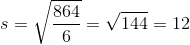Therefore, the answer is that either statement alone is sufficient.

### Example Question #2 : Cubes

A sphere is inscribed inside a cube. What is the volume of the sphere?

Statement 1: The surface area of the cube is 216.

Statement 2: The volume of the cube is 216.

EITHER statement ALONE is sufficient to answer the question.

BOTH statements TOGETHER are insufficient to answer the question.

BOTH statements TOGETHER are sufficient to answer the question, but NEITHER statement ALONE is sufficient to answer the question.

Statement 2 ALONE is sufficient to answer the question, but Statement 1 ALONE is NOT sufficient to answer the question.

Statement 1 ALONE is sufficient to answer the question, but Statement 2 ALONE is NOT sufficient to answer the question.

EITHER statement ALONE is sufficient to answer the question.

Explanation:

The diameter of a sphere inscribed inside a cube is equal to the length of one of the edges of a cube. From either the surface area or the volume of a cube, the appropriate formula can be used to calculate this length. Half this is the radius, from which the formula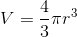can be used to find the volume of the sphere.

### Example Question #1 : Dsq: Calculating The Length Of An Edge Of A Cube

What is the length of edge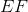of cube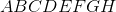(1)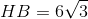.

(2)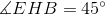.

Statement 2 alone is sufficient.

Statements 1 and 2 together are not sufficient.

Both statements together are sufficient.

Statement 1 alone is sufficient.

Each statement alone is sufficient.

Statement 1 alone is sufficient.

Explanation:

In order to find the length of an edge, we would need any information about one of the faces of the cube or about the diagonal of the cube.

Statement 1 gives us the length of the diagonal of the cube, since the formula for the diagonal is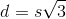whereis the length of an edge of the cube andis the length of the diagonal we are able to find the length of the edge. Therefore statement 1 alone is sufficient.

Statement 2 alones is insufficient, it gives us something we can already tell knowing that ABCDEFGH is a cube.

Statement 1 alone is sufficient.

### Example Question #1 : Dsq: Calculating The Length Of An Edge Of A Cubeis a cube. What is the length of edge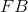?

(1) The volume of the cube is.

(2) The area of faceis.

Statements 1 and 2 together are not sufficient.

Both statements together are sufficient.

Statement 1 alone is sufficient.

Each statement alone is sufficient.

Statement 2 alone is sufficient.

Each statement alone is sufficient.

Explanation:

Like we have previously seen, to find the length of an edge, we need to have information about the other faces or anything else within the cube.

Statement 1 tells us that the volume of the cube is, from this we can find the length of the side of the cube. Statement 1 alone is sufficient.

Statement 2, tells us that the area of ABCD is, similarily, by taking the square root of this number, we can find the length of the edge of the cube.

Therefore each statement alone is sufficient.

### Example Question #1 : Cubes

Find the length of an edge of the cube.

1. The volume of the cube is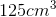.
2. The surface area of the cube is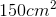.

Statement 2 alone is sufficient, but statement 1 alone is not sufficient to answer the question.

Statement 1 alone is sufficient, but statement 2 alone is not sufficient to answer the question.

Both statements taken together are sufficient to answer the question, but neither statement alone is sufficient.

Statements 1 and 2 are not sufficient, and additional data is needed to answer the question.

Each statement alone is sufficient to answer the question.

Each statement alone is sufficient to answer the question.

Explanation:

Statement 1:  Use the volume formula for a cube to solve for the side length.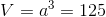whererepresents the length of the edge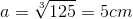Statement 2:   Use the surface area formula for a cube to solve for the side length.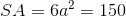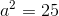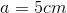Each statement alone is sufficient to answer the question.

### Example Question #1 : Cubesis a cube. What is the length of diagonal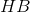?

(1) The area of a face of the cube is.

(2) The length of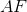is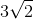.

Statement 2 alone is sufficient.

Statement 1 alone is sufficient.

Each statement alone is sufficient.

Both statements taken together are sufficient.

Statements 1 and 2 taken together are not sufficient.

Each statement alone is sufficient.

Explanation:

To know the diagonal HB of the cube, we need to have information about the length of the edges of the cube. Knowing the area of a face would allow us to find the length of an edge; therefore statement 1 alone is sufficient.

Statment 2 alone is sufficient as well since the length of a diagonal of a square is given by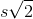, whereis the length of a side of the square and ultimately we can find the length of an edge of the square.

Therefore each statement alone is sufficient.

### Example Question #51 : Rectangular Solids & Cylinders

Calculate the diagonal of a cube.

1. The surface area of the cube is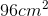.
2. The volume of the cube is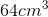.

Both statements taken together are sufficient to answer the question, but neither statement alone is sufficient.

Statements 1 and 2 are not sufficient, and additional data is needed to answer the question.

Statement 1 alone is sufficient, but statement 2 alone is not sufficient to answer the question.

Statement 2 alone is sufficient, but statement 1 alone is not sufficient to answer the question.

Each statement alone is sufficient to answer the question.

Each statement alone is sufficient to answer the question.

Explanation:

Statement 1: We can use the surface area to find the length of the cube's edge and then determine the length of the diagonal.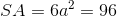whererepresents the length of the cube's edge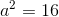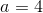Now that we know the length of the edge, we can find the length of the diagonal: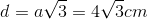.

Statement 2: Once again, we can use the provided information to find the length of the cube's edge.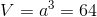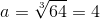Which means the length of the diagonal is.

Thus, each statement alone is sufficient to answer the question.

### Example Question #1 : Cubes

Give the length of the diagonal of the cube.

1. The surface area of the cube is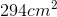.
2. The volume of the cube is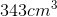.

Statement 2 alone is sufficient, but statement 1 alone is not sufficient to answer the question.

Each statement alone is sufficient to answer the question.

Statement 1 alone is sufficient, but statement 2 alone is not sufficient to answer the question.

Statements 1 and 2 are not sufficient, and additional data is needed to answer the question.

Both statements taken together are sufficient to answer the question, but neither statement alone is sufficient.

Each statement alone is sufficient to answer the question.

Explanation:

Statement 1: To find the length of the diagonal of the cube we need to find the length of the cube's edge. We can use the given surface area value to do so: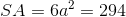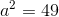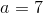Now that we know the length of the cube's edge, we can calculate the diagonal: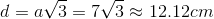Statement 2: We're given the volume of the cube which we can also use to solve for the length of the cube's edge.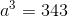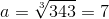Knowing the length of the cube's edge allows us to calculate the diagonal:Each statement alone is sufficient to answer the question.

### Example Question #341 : Data Sufficiency Questions

Find the diagonal of the cube.

1. The surface area of the cube is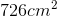.
2. The length of an edge of the cube measures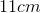.

Statements 1 and 2 are not sufficient, and additional data is needed to answer the question.

Statement 2 alone is sufficient, but statement 1 alone is not sufficient to answer the question.

Each statement alone is sufficient to answer the question.

Both statements taken together are sufficient to answer the question, but neither statement alone is sufficient.

Statement 1 alone is sufficient, but statement 2 alone is not sufficient to answer the question.

Each statement alone is sufficient to answer the question.

Explanation:

Statement 1: We need the length of an edge in order to find the diagonal of the cube. Luckily, we can find the length using the information provided: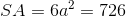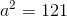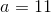Now that we know the length of an edge is 11 cm we can find the length of the diagonal.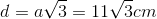Statement 2: We're given the information we need to find the diagonal, all we need to do is plug it into the equation### Example Question #10 : Cubes

What is the length of the diagonal of the cube A if the diagonal of cube B is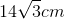?

1. The lengths of the edges of cube A to cube B is a ratio of 1:2.
2. The surface area of cube A is.

Both statements taken together are sufficient to answer the question, but neither statement alone is sufficient.

Statement 2 alone is sufficient, but statement 1 alone is not sufficient to answer the question.

Statements 1 and 2 are not sufficient, and additional data is needed to answer the question.

Each statement alone is sufficient to answer the question.

Statement 1 alone is sufficient, but statement 2 alone is not sufficient to answer the question.

Each statement alone is sufficient to answer the question.

Explanation:

Statement 1: The information provided in the question is only useful if we're given a relationship between cube A and cube B. Since this statement does provide us with the ratio of 1:2, we can answer the question.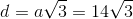whererepresents the length of the cube's edge

we can easily see the length measures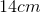Remember the ratio of cube A to cube B is 1:2.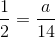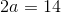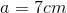Now that we know the length, we can find the diagonal of the cube: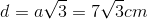Statement 2: We're given information about cube A so we don't need to worry about cube B. Using this information we can solve for the edge length of cube A and then calculate the diagonal.Knowing the length of the edge allows us to find the diagonal of the cube← Previous 1

### All GMAT Math Resources